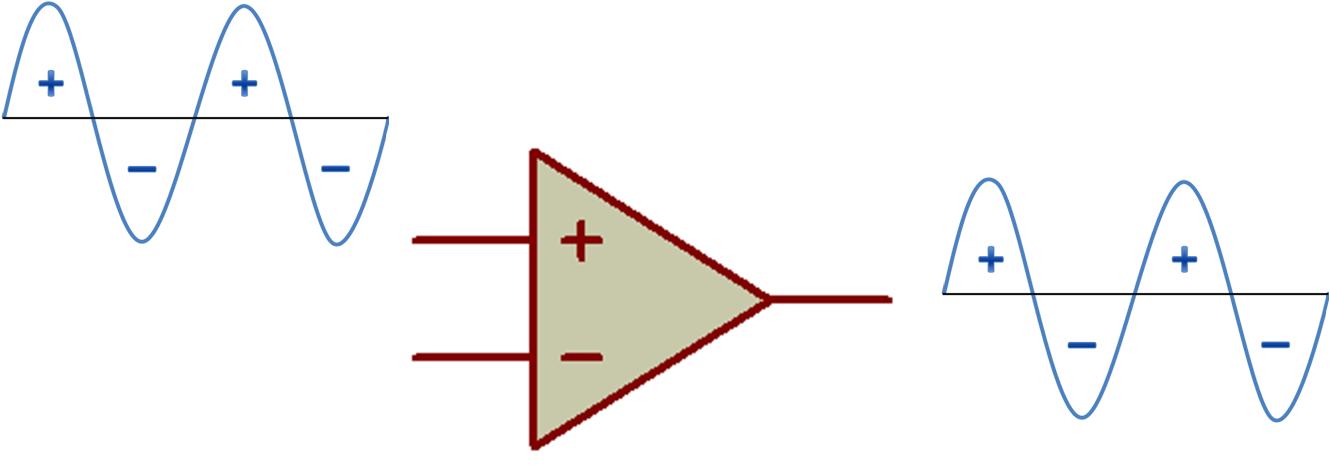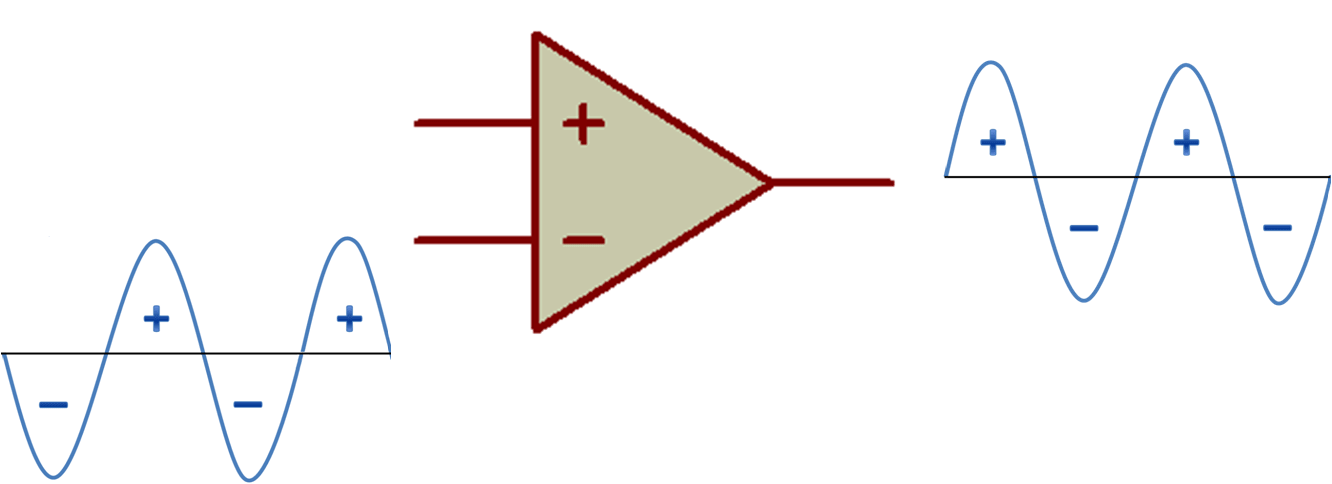# OPAMP OR OPERATIONAL AMPLIFIER

In this tutorial section we will discuss about input and output signals of operational amplifier or OPAMP and OPAMP ICs which includes LM741 and LM358.

## OPERATIONAL AMPLIFIER

Operational amplifier is a DC coupled high gain voltage amplifier with a differential input and whose output is several hundreds of times of its differential input. In simple terms, sensors give a low voltage output that cannot be easy to read, so an operational amplifier is used to amplify the signal.

Two commonly used opamp are LM741 & LM358. Difference between LM358 & LM741 is, LM358 is newer and have two OP-AMP on chip while in 741 only one OP-AMP is present. Both the IC’s have 8 pins.

In op-amp LM741 Offset is used because any sensor can give output without any input. To avoid noise signals we connect a variable resistor (POT-Potentiometer) in between +Offset and –Offset. Diagrams of the IC are shown below –

## OPAMP WORKING

Op-amp has two inputs namely inverting (–) and non-inverting (+) terminal and one output terminal.

### NON INVERTING (+) INPUT

If the signal is passed through the non-inverting (+) terminal of the op-amp then there would be no change in output signal.### INVERTING (–) INPUT

If the signal is passed through the inverting (–) terminal of the op-amp then output signal will be inverted (just opposite). This is the same as the mathematical concept [+ multiply by number = number], [– multiply by number = – (number)].As op-amp has differential input, this means it compares the strength of the input signals at inverting (–) and non-inverting (+) terminal which one is greater will be considered as input.

NEXT POST
LOGIC GATES USING SWITCH

PREVIOUS POST
TRANSFORMER

Subscribe
Notify of1 Comment
Inline Feedbacks